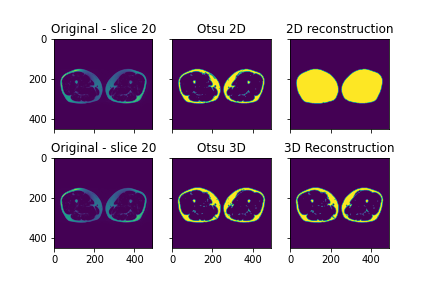# BinaryReconstructionByErosion works on 2D but not 3D images

Hi, I guess this is indeed a beginner question, but here I go.

I have a series of DICOM files of a Dixon sequence of the thigh. My first very simple task is to create a mask of the whole thigh against the background.

To do so at first I read a sitk.Image from DICOM and I use OtsuTreshold to get the outline of the thigh, then I use BinaryReconstructionByErosion in order to fill the outline and have the full mask.

This works fine when I do it in 2D (i.e. opening one DICOM at a time and processing it).

However, if I try to do it on a 3D image obtained by reading the entire DICOM series, the process fails, and instead of having a filled mask as output of the reconstruction I have a mask that is identical to the Otsu mask calculated in the first step (cfr plot below).

HereHere the code that I used:

``````from os import chdir
import SimpleITK as sitk
from glob import glob
import matplotlib.pyplot as plt
import numpy as np
from skimage.morphology import reconstruction

def copy_sitk_space_info(templateImage, newImage):
newImage.SetOrigin(templateImage.GetOrigin())
newImage.SetDirection(templateImage.GetDirection())
newImage.SetSpacing(templateImage.GetSpacing())
return(newImage)

return(inputImage)

#input file
input_dcm_dir = "/my/dcm/dir"
chdir(input_dcm_dir)
f = glob("*")
# 2D processing
maskImage = sitk.OtsuThreshold( im, 0, 1, 200 )
#initalise seed image
seedImage = sitk.GetImageFromArray(seed_image)
#3D processing
inputImage = sitk.Cast(inputImage, sitk.sitkFloat32)
maskImage_3D = sitk.OtsuThreshold( inputImage, 0, 1, 200 )
#initalise seed image
seedImage = sitk.GetImageFromArray(seed_image)

fig, axs = plt.subplots(2, 3)
axs[0, 0].imshow((sitk.GetArrayFromImage(im).squeeze()))
axs[0, 0].set_title('Original - slice 20')
axs[0, 1].set_title('Otsu 2D')
axs[0, 2].imshow((sitk.GetArrayFromImage(filledImage).squeeze()))
axs[0, 2].set_title('2D reconstruction')
axs[1, 0].imshow((sitk.GetArrayFromImage(im).squeeze()))
axs[1, 0].set_title('Original - slice 20')
axs[1, 1].set_title('Otsu 3D')
axs[1, 2].imshow((sitk.GetArrayFromImage(filledImage3D).squeeze()[20,:,:]))
axs[1, 2].set_title('3D Reconstruction')
for ax in axs.flat:
ax.label_outer()
plt.savefig("2D_3D_erosion.png")
``````

I guess that my main question is: am I doing something wrong or does indeed the BinaryReconstructionByErosion only work on 2D images ? And if this is the case, what it the best and more adapted method to apply an operation to several slices of a 3D image and get back the output as another 3D image ?

Here you’ll find an archive with the original DICOM files that I used.

Thank in advance for any tips or suggestion

The filter is working correctly.

The segmented thighs are open at either end, so the erosion of the mask image occurs from those open ends.

I don’t see a problem doing this operation on a series of 2d slices.

Here is some example code of doing slice-by-slice operations in python with SimpleITK:

1 Like

Thanks a lot for the clarification and the link to the other discussion !
Of course now that you pointed out that the thighs mask is open at the two extremities I feel like a complete noob (that I am) for ignoring that.